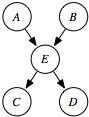### 11.2.1 Learning the Probabilities

The simplest case is when we are given the structure of the model and all of the variables have been observed. In this case, we must learn only the probabilities. This is very similar to the case of learning probabilities in Section 7.3.3.

Model Data ProbabilitiesA B C D E t f t t f f t t t t t t f t f ···
 P(A) P(B) P(E|A,B) P(C|E) P(D|E)
Figure 11.5: From the model and the data, learning the probabilities

Each conditional probability distribution can be learned separately using the empirical data and pseudocounts or in terms of the Dirichlet distribution.

Example 11.4: Figure 11.5 shows a typical example. We are given the model and the data, and we must infer the probabilities.

For example, one of the elements of P(E|AB) is

 P(E=t | A=t ∧B=f) = ((# examples: E=t ∧A=t ∧B=f) + c1)/((# examples: A=t ∧B=f) + c) ,

where c1 is a pseudocount of the number of cases where E=t ∧A=t ∧B=f, and c is a pseudocount of the number of cases where A=t ∧B=f. Note that c1 ≤ c.

If a variable has many parents, using the counts and pseudo counts can suffer from overfitting. Overfitting is most severe when there are few examples for some of the combinations of the parent variables. In that case, the techniques of Chapter 7 can be used: for example, learning decision trees with probabilities at the leaves, sigmoid linear functions, or neural networks. To use supervised learning methods for learning conditional probabilities of a variable X given its parents, the parents become the input nodes and X becomes the target feature. Decision trees can be used for arbitrary discrete variables. Sigmoid linear functions and neural networks can represent a conditional probability of a binary variable given its parents. For non-binary variables, indicator variables can be used.